## 7.5Wall functions

CFD simulations may be used to calculate the forces on solid bodies exerted by the ﬂuid, e.g. in aerodynamics. The wall shear stress is then calculated according to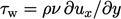. With turbulent boundary layers, the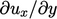calculation requires cells with very small lengths normal to the wall to be accurate. The resulting mesh is inevitably large, which carries a high computational cost. The problem for CFD is how to calculate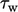with suﬃcient accuracy, but at an aﬀordable cost.

Wall functions provide a solution to this problem by exploiting the universal character of the velocity distribution described in Sec. 7.4 . They use the law of the wall Eq. (7.13 ) as a model to provide a reasonable prediction of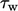from a relatively inaccuratecalculation at the wall.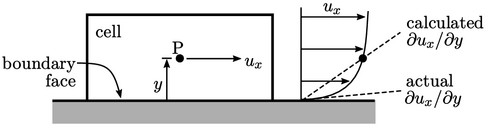Wall functions use the near-wall cell centre height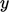, i.e. the distance to the wall from the centre P of each near-wall cell. Typically when using wall functions,should correspond to a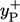within the typical range of applicability of the log law Eq. (7.13 ), i.e.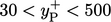.

With such a mesh, the calculated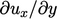is then signiﬁcantly lower than its true value. Wall function models compensate for the resulting error in the prediction of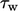by increasing viscosity at the wall. The increase is applied to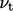at the wall patch faces, which would otherwise be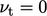, corresponding to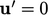.

### Standard wall function

The standard wall function for a “smooth” wall calculates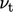for each patch face based on the near-wall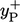. No adjustment is made to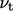whencorresponds to the viscous sub-layer. Whencorresponds to the inertial sub-layer,is calculated by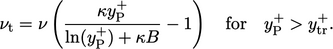(7.17)
The condition (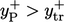) that determines whetherlies within the inertial sub-layer corresponds to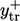at the intersection of Eq. (7.11 ) and Eq. (7.13 ), calculated iteratively as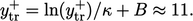(7.18)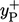is calculated in each near-wall cell according to: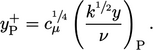(7.19)
This expression is derived from Eq. (7.9 ) for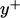and Eq. (7.16 ). The subscript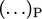denotes all properties are evaluated at P.

The wall function Eq. (7.17) is derived from the notion that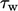is calculated numerically (assuming a stationary wall) by: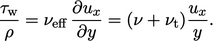(7.20)
At the same time, combining Eq. (7.9 ) and Eq. (7.10 ) gives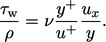(7.21)
Comparing Eq. (7.20 ) and Eq. (7.21 ) gives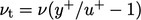, which combines with Eq. (7.13 ) to yield Eq. (7.17).
Notes on CFD: General Principles - 7.5 Wall functions